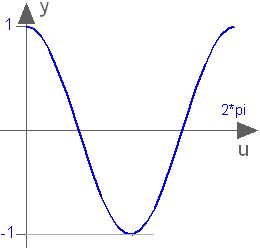# 'cos()'

cos()# Information

This information is part of the Modelica Standard Library maintained by the Modelica Association.

Trigonometric cosine function

`cos(u)`

#### Description

Returns the cosine of u, with -∞ < u < ∞ Argument u needs to be an Integer or Real expression.

The cosine function can also be accessed as Modelica.Math.cos.#### Examples

```cos(3.14159265358979)
= -1.0```